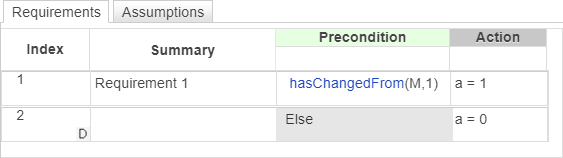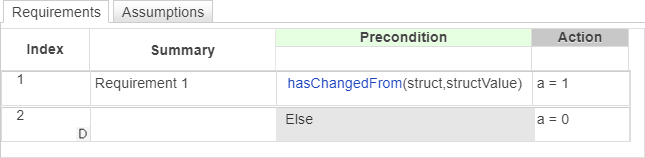# hasChangedFrom

Detect change in data from specified value

## Syntax

``tf = hasChangedFrom(data,value)``

## Description

example

````tf = hasChangedFrom(data,value)` returns `1` (`true`) if the value of `data` is equal to `value` at the beginning of the previous time step and is a different value at the beginning of the current time step. Otherwise, the operator returns `0` (`false`). Use this operator in the Requirements Table block.```

## Examples

expand all

Set the output data `a` to `1` if the input data `M` has changed from `1` since the last time step. Otherwise, set `a` to `0`.Set the output data `a` to `1` if the element in row 1 and column 3 of input data `M` has changed from `1` since the last time step. Otherwise, set `a` to `0`.Set the output data `a` to `1` if one of the fields of the structure `struct` has changed from the value of `structValue` since the last time step. Otherwise, set `a` to `0`.Set the output data `a` to `1` if the field `struct.field` has changed from the value of `1` since the last time step. Otherwise, set `a` to `0`.## Input Arguments

expand all

Data defined in the Requirements Table block, specified as a:

• Scalar

• Matrix or an element of a matrix

• Structure or a field in a structure

• Valid combination of structure fields or matrix elements

If `data` is a matrix, the operator returns `true` when it detects a change in one of the elements of `data`. You can also index elements of a matrix by using numbers or expressions that evaluate to a integer.

If `data` is a structure, the operator returns `true` when it detects a change in one of the fields of `data`. You can also index fields in a structure by using dot notation.

The argument `data` cannot be a nontrivial expression or a custom code variable.

Data Types: `single` | `double` | `int8` | `int16` | `int32` | `int64` | `uint8` | `uint16` | `uint32` | `uint64` | `logical` | `string` | `fi` | `enumerated` | `bus`

Value of the data at previous time step, specified as the same data type of `data`. `value` must be an expression that resolves to a value that is comparable with `data`:

• If `data` is a scalar, then `value` must resolve to a scalar.

• If `data` is a matrix, then `value` must resolve to a matrix with the same dimensions as `data`.

• If `data` is a structure, then `value` must resolve to a structure whose field specification matches `data` exactly.

Data Types: `single` | `double` | `int8` | `int16` | `int32` | `int64` | `uint8` | `uint16` | `uint32` | `uint64` | `logical` | `string` | `fi` | `enumerated` | `bus`

## Tips

• If the Requirements Table block writes to the specified data but does not change the value, the `hasChangedFrom` operator returns `false`.

## Version History

Introduced in R2022a